Introduction to Multiplication

 Problem Set 1 Problem Set 2 Problem Set 3Select a problem set using the buttons above, then use your mouse or tab key to select a question. Fill in the blank with the correct answer for each problem. When you have answered all of the questions, ask Charlie how you did.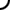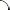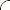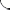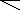Perform each multiplication operation. Be sure to use commas where appropriate.   6 • 7 = 5 • 9 = 8 • 12 = 12 • 11 = 30 • 7 = 154 • 13 =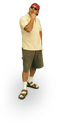Perform each multiplication operation. Be sure to use commas where appropriate.   7 • 7 = 6 • 9 = 8 • 13 = 12 • 12 = 30 • 5 = 154 • 12 =Perform each multiplication operation. Be sure to use commas where appropriate.   8 • 7 = 7 • 9 = 8 • 11 = 12 • 13 = 30 • 6 = 154 • 11 =©  2009  LarryPerezWeb Design:  Patrick Quigley